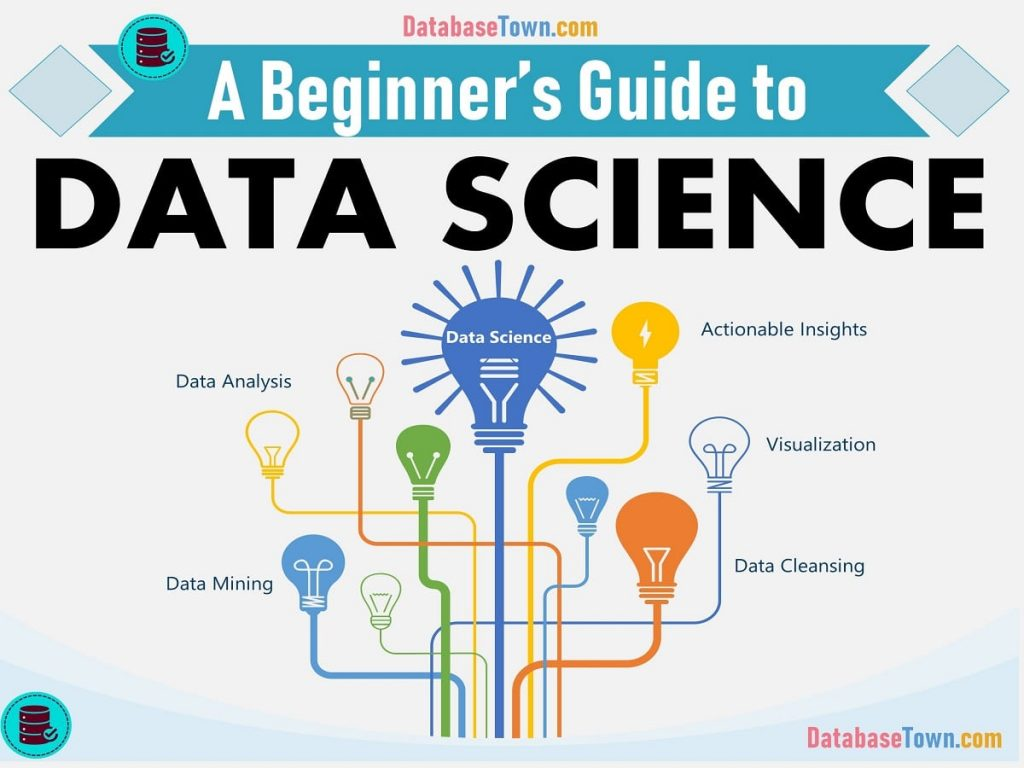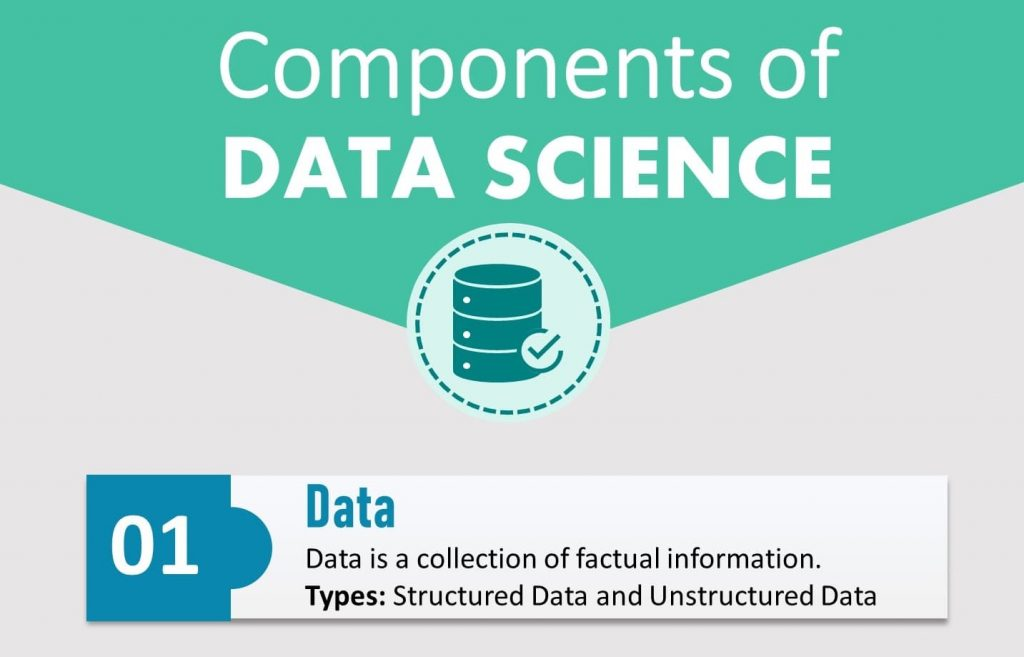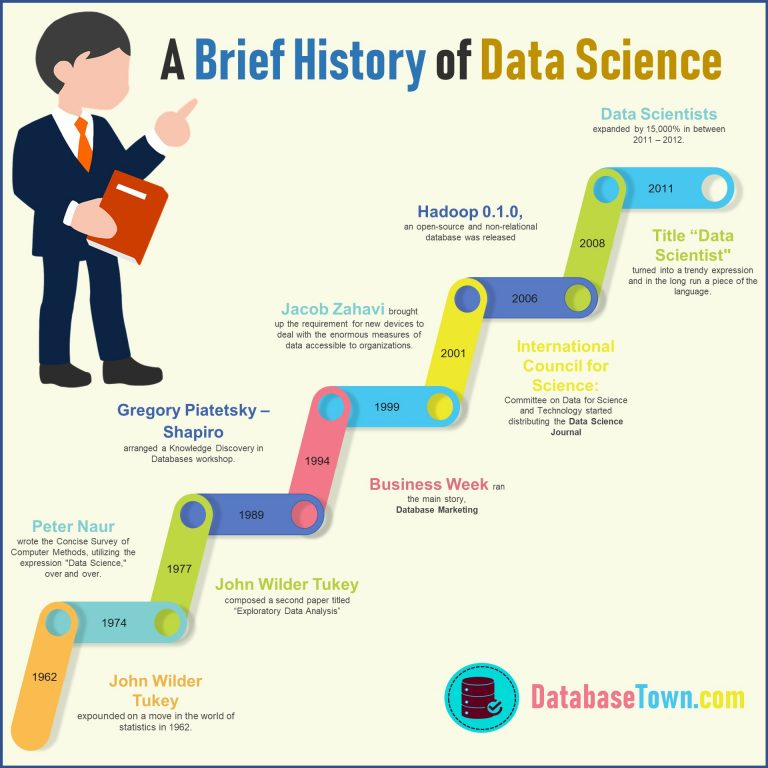## Step-by-Step Introduction to Data Science | A Beginner’s Guide

Welcome to the tutorial of data science. This is beginner’s guide on introduction to data science. There are different sections in this guide. Each section will help you to clear the basic concepts of data science. Let’s start! Introduction to Data Science In present era, the term Data Science is frequently being used but every …## 5 Basic Components of Data Science

Data science consists of many algorithms, theories, components etc. Before detail study of data science, we need to understand them. Five basic components of data science are discussed here. 1. Data Data is a collection of factual information based on numbers, words, observations, measurements which can be utilized for calculation, discussion and reasoning. The crude …## A Brief History of Data Science

Before proceeding to the introduction, a brief history of data science is described here that will enable you to understand how data science evolved and from where it started. Historical background of Data Science Data originates from the Latin word, “datum,” which means a “something given” The expression “data” has been utilized since 1500s, however, …